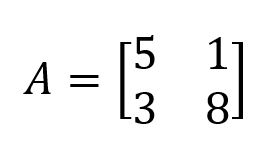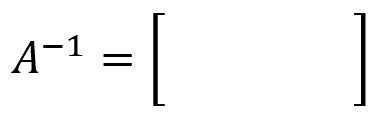Robotics 2
Path-Planning and Trajectory Generation
QuizQuestion 2:  In a path equation, what do we want the 'parameter' to represent?
Question 3:  When we write an equation to calculate the joint velocities given the end-effector velocities, what do we have to do with the Jacobian matrix?
Questions 4-7:  Suppose I have a 2x2 matrix as shown here.  Fill in the values of the inverse of the matrix.  Round to two decimal places.
Question 8:  True or false: we can only calculate the inverse of a matrix if it is square.
Question 9:  If I have a manipulator with 3 joints, how many of the components of the end-effector velocity can I control simultaneously?
Question 1: When we define a path of the end-effector to take between two end-points, what kind of equation is most useful?
Question 10:  Once I have defined the path I want the end-effector to follow, how do I find the end-effector velocity (the trajectory)?
A parametric equation
An inverse equation
A parabolic equation
Time
Velocity
Distance
Acceleration
Take the inverse
Take the transpose
Take the derivative
Take the integralTrue
False
All 6
At least 3
Only 3
Fewer than 3
Take the derivative
Take the integral
Take the inverse
Take the transpose